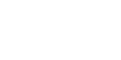The elements used in the geometric application, can be divided into two groups: simple and complex. Rectangles, parallelograms, isosceles trapezoids, isosceles triangles make up the first group of figures (figure 4.1). This is the basic form, which based the composition in a geometric application. Masters used, and more complex geometric shapes (the second group). These include irregular triangles and quadrilaterals, right and wrong polygons with convex and concave corners (figure 4.2).First consider how from a straw strips are simple geometric elements. Then consider the methods by which it is possible to obtain more complex geometrical elements.
Simple shapes. To obtain identical squares straw tape is cut with a knife-a joint perpendicular to the fibers (figure 4.3 a, b). The hand will learn to "remember" dimensions as will accumulate experience.
Elements of rectangular shape are obtained when the same position of the cutter, as when making a square. The only difference is that changing the aspect ratio: the rectangle they are not equal (figure 4.3 a, b).
The elements in the form of a parallelogram cut from a straw of the tape when the angular position of the knife blade is joint to the fibers (figure 4.4). If you are running a large number of identical parallelograms, one should look to every slice was parallel to the previous one. If you change the longitudinal size of the parallelograms, they will get different proportions: from elongated to oval. By changing the position of the blade relative to the fiber also changes the shape of parallelograms. With a smaller angle, the elements are more elongated and pointed and dynamic. If the angle is closer to 90°, the parallelogram is closer to a rectangle.
Elements in the shape of trapezoids cut off from the straw tape while alternating the position of the knife blade to the right and left (figure 4.5). When you run this item, you should pay attention to the corners by changing the position of the blade in different directions was equal. If you change the longitudinal size of the trapezoid and angular position of the blade is possible to obtain different forms of trapezoids.Elements in the form of isosceles and equilateral triangles can be obtained in two ways. The first method (figure 4.6) allows us to obtain the triangles of the above mentioned types with any angle at the vertex. This method is similar to the method of formation of the trapezoids. Line-cut must intersect at a point located on the edge of the tape (figure 4.6). When you angle the blade right and left, the amount of tilt should remain the same.
To obtain a rectangular equilateral triangles, elements, cut off two positions of the cutter: first position at an angle of 45°, the second at 90° to the direction of the straw fibers of the ribbon (figure 4.7).
To obtain rectangular triangles with a different aspect ratio, the angle at the first position of the cutter is replaceable for different from 45°.
Complex shapes. The shapes of the second group is more difficult to systematize the ways of formation of straw ribbons. It is possible to give some General recommendations to help further their own.
Of squares and other rectangles you can get correct and incorrect polygons. Parallelograms and trapezoids can be the basis for formation of a variety of triangles (figure 4.8).
Constituent elements. If you need the element has a complex shape and it is impossible to obtain immediately from straw ribbon in any of the above methods, you can try to compose it from several simple elements (figure 4.9, 4.10, 4.11, 4.12, 4.13). In the drawings, the variants of the formation of the constituent elements obtained on the basis of rectangles, parallelograms, trapezoids, triangles. Thanks to the various combinations of simple and complicated geometries it is possible to countless options of ornamental motifs. In the development of the individual motive is very important to consider proportion and proportionality (more, less) used elements relative to each other. Due to its texture straw while covering its fibers at different angles changes the shades from light to dark, depending on, the rays fall parallel to the fibers or perpendicular to them. Therefore, to obtain additional colour effects in the compositions used components. How is it done and how to achieve the effects of convexity and concavity using a different orientation of straw fibers in the elements when placing them on the plane, we'll talk further.